Skip to content

# ODE Analysis

## Analysis of an Ordinary Differential Equation (ODE)

All the figures below are generated using examples/ode/ode.jl.

### ODE

$$x''(t) = -\omega^2 * x(t) - k * x'(t)$$

#### Unknown ODE parameters

• k
• $\omega$

#### Example ODE solutionFor model parameters:

• k = 0.1
• $\omega$ = 0.2

### Local sensitivity analysis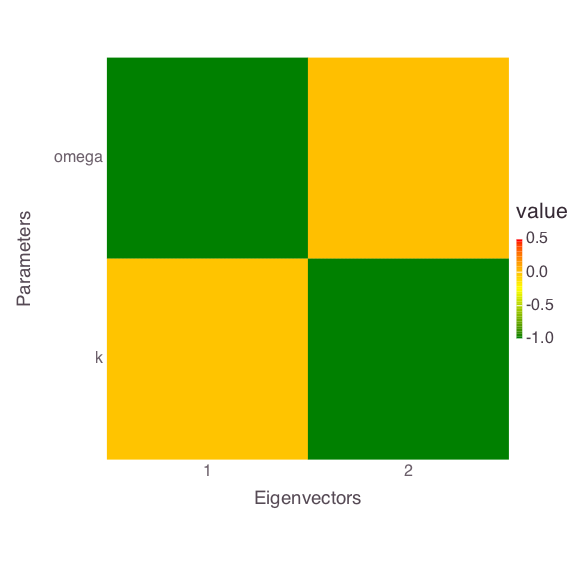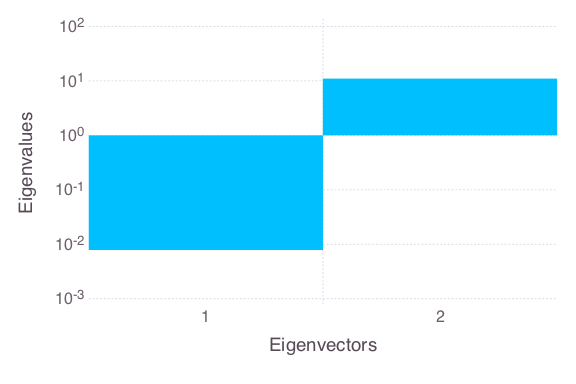### Global sensitivity analysis (using eFAST)Probabilistic distributions of the prior parameter uncertainties are:

• k = LogUniform(0.01, 0.1)
• $\omega$ = Uniform(0.1, 0.3)

### Bayesian sensitivity analysis

#### Observations

Synthetic observations are applied to constrain the ODE parameters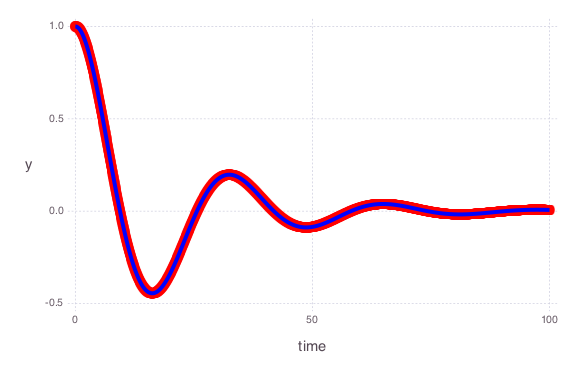Observation errors are equal for all the sample locations with standard deviation equal to 1 (observation weight = 1 / observation standard deviation = 1 / 1 = 1)

#### Prior parameter uncertainties

##### k only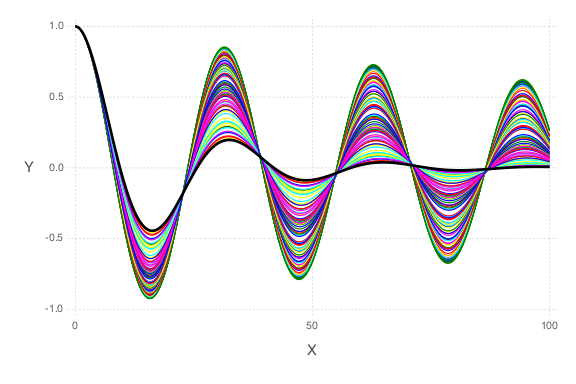##### $\omega$ only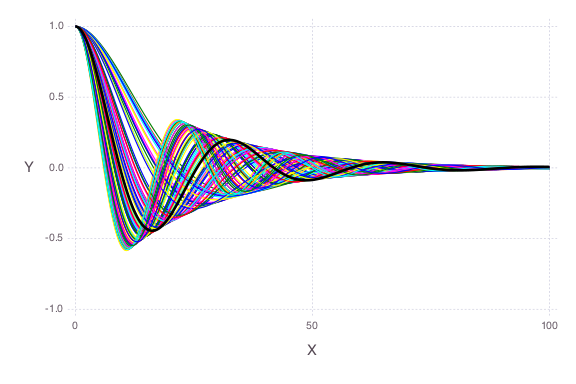##### Both parameters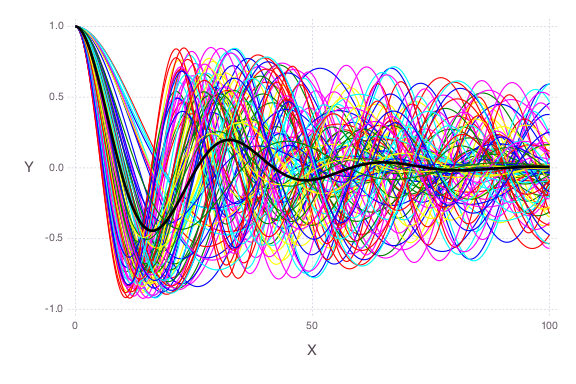The observation data are plotted as a solid black line.

#### Histograms/scatter plots of Bayesian MCMC results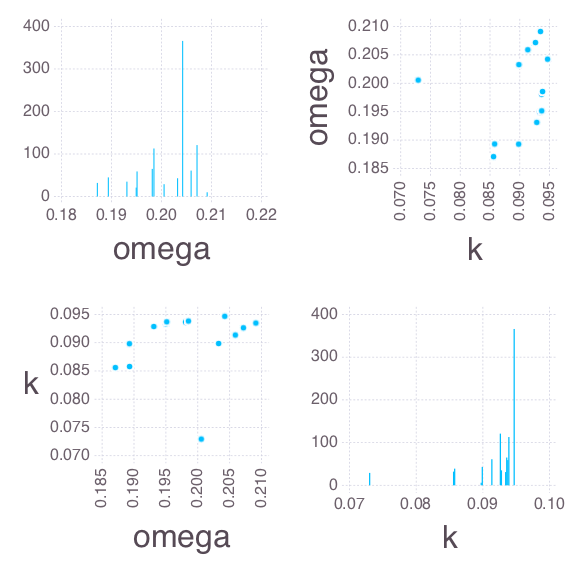#### Posterior parameter uncertainties

Note that now the parameter uncertainties are constrained by the observation data.

##### k only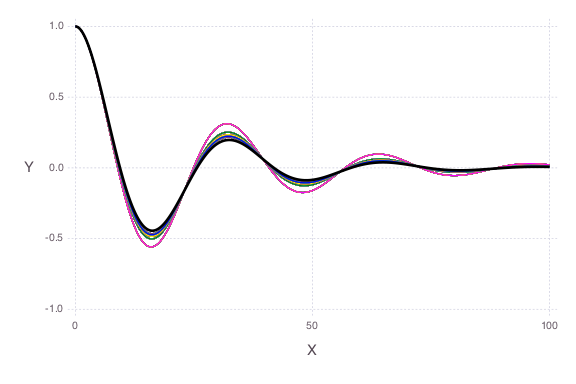##### $\omega$ only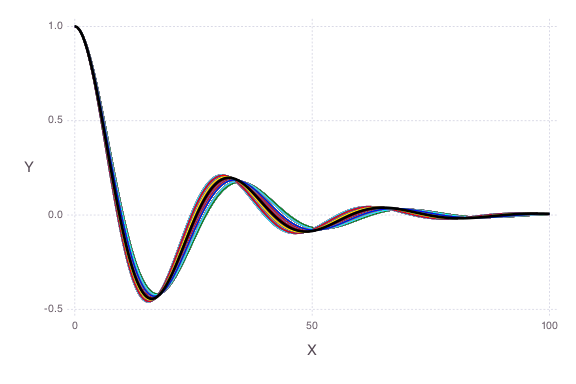##### Both parameters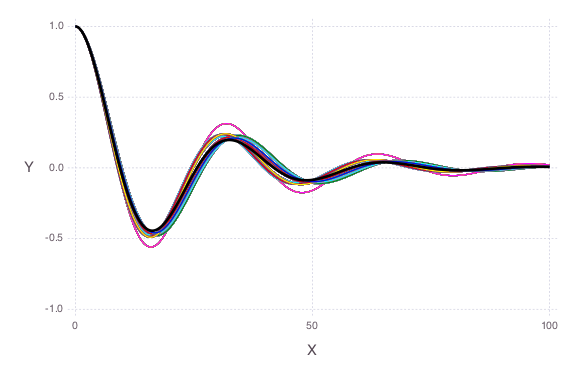The observation data are plotted as a solid black line.